# Search Results (54)

View
Selected filters:
• MCCRS.Math.Content.7.EE.B.4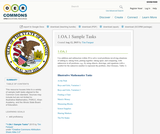Conditions of Use:
Remix and Share
Rating

This resource houses links to a variety of sample math tasks aligned to the Common Core standard. Sources may include but are not limited to: Illustrative Mathematics, PARCC, Khan Academy, and the Illinois State Board of Education.

Subject:
Mathematics
Material Type:
Assessment
Homework/Assignment
Lecture
Teaching/Learning Strategy
Author:
Tim Farquer
01/28/2016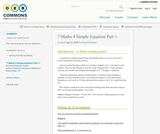Conditions of Use:
Remix and Share
Rating

This resource gives intro to equations

Subject:
Mathematics
Material Type:
Author:
Peeyush Kumar
01/28/2016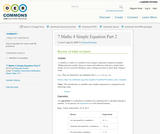Conditions of Use:
Remix and Share
Rating

Use of equation for some real life problems.

Subject:
Mathematics
Material Type:
Author:
Peeyush Kumar
01/28/2016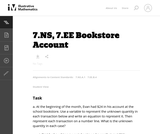Conditions of Use:
No Strings Attached
Rating

This is a task from the Illustrative Mathematics website that is one part of a complete illustration of the standard to which it is aligned. Each task has at least one solution and some commentary that addresses important asects of the task and its potential use. Here are the first few lines of the commentary for this task: At the beginning of the month, Evan had \24 in his account at the school bookstore. Use a variable to represent the unknown quantity in each transacti... Subject: Mathematics Material Type: Activity/Lab Provider: Illustrative Mathematics Provider Set: Illustrative Mathematics Author: Illustrative Mathematics Date Added: 05/13/2013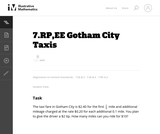Conditions of Use: No Strings Attached Rating This is a task from the Illustrative Mathematics website that is one part of a complete illustration of the standard to which it is aligned. Each task has at least one solution and some commentary that addresses important asects of the task and its potential use. Here are the first few lines of the commentary for this task: The taxi fare in Gotham City is \2.40 for the first $\frac{1}{2}$ mile and additional mileage charged at the rate \\$0.20 for each additional 0.1 mile....

Subject:
Mathematics
Material Type:
Activity/Lab
Provider:
Illustrative Mathematics
Provider Set:
Illustrative Mathematics
Author:
Illustrative Mathematics
03/17/2013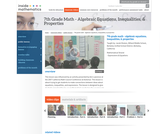Conditions of Use:
Rating

This lesson is about trying to get students to make connections between ideas about equations, inequalities, and expressions. The lesson is designed to give students opportunities to use mathematical vocabulary for a purpose to describe, discuss, and work with these symbol strings.The idea is for students to start gathering global information by looking at the whole number string rather than thinking only about individual procedures or steps. Hopefully students will begin to see the symbol strings as mathematical objects with their own unique set of attributes. (7th Grade Math)

Subject:
Algebra
Numbers and Operations
Material Type:
Activity/Lab
Lecture
Lesson Plan
Teaching/Learning Strategy
Provider:
Noyce Foundation
Provider Set:
Inside Mathematics
Author:
Disston, Jacob
11/30/2011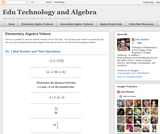Conditions of Use:
Remix and Share
Rating

Visually searchable database of Algebra 1 videos. Click on a problem and watch the solution on YouTube. Copy and paste this material into your CMS. Videos accompany the open Elementary Algebra textbook published by Flat World Knowledge.

Subject:
Algebra
Material Type:
Lecture
Lecture Notes
Provider:
Individual Authors
Provider Set:
Individual Authors
Author:
John Redden
04/29/2012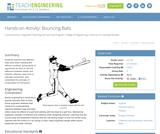Conditions of Use:
Rating

Students examine how different balls react when colliding with different surfaces, giving plenty of opportunity for them to see the difference between elastic and inelastic collisions, learn how to calculate momentum, and understand the principle of conservation of momentum.

Subject:
Engineering
Physics
Material Type:
Activity/Lab
Provider:
TeachEngineering
Provider Set:
TeachEngineering
Author:
Bailey Jones
Chris Yakacki
Denise Carlson
Malinda Schaefer Zarske
Matt Lundberg
10/14/2015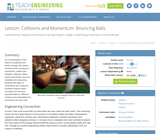Conditions of Use:
Rating

As a continuation of the theme of potential and kinetic energy, this lesson introduces the concepts of momentum, elastic and inelastic collisions. Many sports and games, such as baseball and ping-pong, illustrate the ideas of momentum and collisions. Students explore these concepts by bouncing assorted balls on different surfaces and calculating the momentum for each ball.

Subject:
Engineering
Physics
Material Type:
Activity/Lab
Lesson Plan
Provider:
TeachEngineering
Provider Set:
TeachEngineering
Author:
Bailey Jones
Chris Yakacki
Denise Carlson
Malinda Schaefer Zarske
Matt Lundberg
09/18/2014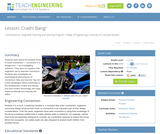Conditions of Use:
Rating

Students learn about the physical force of linear momentum movement in a straight line by investigating collisions. They learn an equation that engineers use to describe momentum. Students also investigate the psychological phenomenon of momentum; they see how the "big mo" of the bandwagon effect contributes to the development of fads and manias, and how modern technology and mass media accelerate and intensify the effect.

Subject:
Engineering
Physics
Material Type:
Activity/Lab
Lesson Plan
Provider:
TeachEngineering
Provider Set:
TeachEngineering
Author:
Ben Heavner
Chris Yakacki
Denise Carlson
Malinda Schaefer Zarske
09/18/2014Conditions of Use:
Rating

In this two-part activity, students design and build Rube Goldberg machines. This open-ended challenge employs the engineering design process and may have a pre-determined purpose, such as rolling a marble into a cup from a distance, or let students decide the purposes.

Subject:
Engineering
Material Type:
Activity/Lab
Provider:
TeachEngineering
Provider Set:
TeachEngineering
Author:
Janet Yowell
Michael J. Bendewald
10/14/2015Rating

This lesson unit is intended to help assess how well students are able to interpret and use scale drawings to plan a garden layout. This involves using proportional reasoning and metric units.

Subject:
Algebra
Geometry
Ratios and Proportions
Material Type:
Assessment
Lesson Plan
Provider:
Shell Center for Mathematical Education
U.C. Berkeley
Provider Set:
Mathematics Assessment Project (MAP)
Mathematics Assessment Project (MAP)
04/26/2013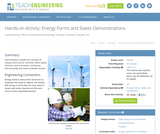Conditions of Use:
Rating

Demonstrations explain the concepts of energy forms (sound, chemical, radiant [light], electrical, atomic [nuclear], mechanical, thermal [heat]) and states (potential, kinetic).

Subject:
Engineering
Physics
Material Type:
Activity/Lab
Provider:
TeachEngineering
Provider Set:
TeachEngineering
Author:
Jan DeWaters
Susan Powers
09/18/2014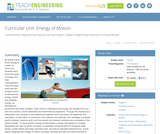Conditions of Use:
Rating

By taking a look at the energy of motion all around us, students learn about the types of energy and their characteristics. They first learn about the two simplest forms of mechanical energy: kinetic and potential energy, as illustrated by pendulums and roller coasters. They come to understand that energy can change from one form into another, and be described and determined by equations. Through the example of a waterwheel, the concepts of and differences between work and power are explained and calculated. Conservation of momentum and collisions are explored, with analogies to popular sports (billiards, baseball, golf), and how elastic and inelastic collisions are considered in the games' design. To show another energy transformation concept, the behavior of energy dissipating into heat by means of friction is presented. Students learn to recognize static friction, kinetic friction and drag, how they work, and how to calculate frictional force. A final lesson integrates the energy of motion concepts, showing how they are interconnected in everyday applications such as skateboards, scooters, roller coasters, trains, cars, planes, trucks and elevators. Through numerous hands-on activities, students swing pendulums, use plastic two-liter bottles to construct model waterwheels, bounce different types of balls, use weights to generate friction data, and roll balls down ramps to collide into cups.

Subject:
Engineering
Physics
Material Type:
Full Course
Provider:
TeachEngineering
Provider Set:
TeachEngineering
10/14/2015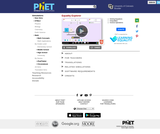Conditions of Use:
No Strings Attached
Rating

Explore what it means for a mathematical statement to be balanced or unbalanced by interacting with objects on a balance. Discover the rules for keeping it balanced. Collect stars by playing the game!

Subject:
Mathematics
Material Type:
Simulation
Provider:
Provider Set:
PhET Interactive Simulations
Author:
David Wood
Marleene Buttice
Meenakshi Raghavan
03/06/2019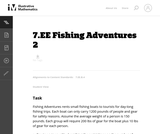Conditions of Use:
No Strings Attached
Rating

This task is the second in a series of three tasks that use inequalities in the same context at increasing complexity in 6th grade, 7th grade and in HS algebra. Students write and solve inequalities, and represent the solutions graphically.

Subject:
Mathematics
Algebra
Material Type:
Activity/Lab
Provider:
Illustrative Mathematics
Provider Set:
Illustrative Mathematics
Author:
Illustrative Mathematics
05/01/2012Conditions of Use:
Rating

The purpose of this lesson is to teach the students about how a spacecraft gets from the surface of the Earth to Mars. The lesson first investigates rockets and how they are able to get us into space. Finally, the nature of an orbit is discussed as well as how orbits enable us to get from planet to planet specifically from Earth to Mars.

Subject:
Engineering
Astronomy
Material Type:
Activity/Lab
Lesson Plan
Provider:
TeachEngineering
Provider Set:
TeachEngineering
Author:
Chris Yakacki
Daria Kotys-Schwartz
Geoffrey Hill
Janet Yowell
Malinda Schaefer Zarske
09/18/2014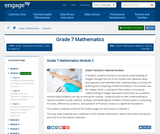Conditions of Use:
Remix and Share
Rating

In Grade 6, students formed a conceptual understanding of integers through the use of the number line, absolute value, and opposites and extended their understanding to include the ordering and comparing of rational numbers. This module uses the Integer Game: a card game that creates a conceptual understanding of integer operations and serves as a powerful mental model students can rely on during the module.  Students build on their understanding of rational numbers to add, subtract, multiply, and divide signed numbers. Previous work in computing the sums, differences, products, and quotients of fractions serves as a significant foundation.

Subject:
Numbers and Operations
Material Type:
Module
Provider:
New York State Education Department
Provider Set:
EngageNY
09/25/2013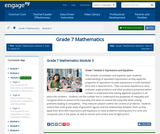Conditions of Use:
Remix and Share
Rating

This module consolidates and expands upon students understanding of equivalent expressions as they apply the properties of operations to write expressions in both standard form and in factored form.  They use linear equations to solve unknown angle problems and other problems presented within context to understand that solving algebraic equations is all about the numbers.  Students use the number line to understand the properties of inequality and recognize when to preserve the inequality and when to reverse the inequality when solving problems leading to inequalities.  They interpret solutions within the context of problems.  Students extend their sixth-grade study of geometric figures and the relationships between them as they apply their work with expressions and equations to solve problems involving area of a circle and composite area in the plane, as well as volume and surface area of right prisms.

Subject:
Algebra
Material Type:
Module
Provider:
New York State Education Department
Provider Set:
EngageNY
11/15/2013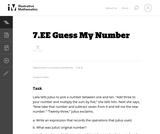Conditions of Use:
No Strings Attached
Rating

This problem asks the students to represent a sequence of operations using an expression and then to write and solve simple equations. The problem is posed as a game and allows the students to visualize mathematical operations. It would make sense to actually play a similar game in pairs first and then ask the students to record the operations to figure out each other's numbers.

Subject:
Mathematics
Algebra
Material Type:
Activity/Lab
Provider:
Illustrative Mathematics
Provider Set:
Illustrative Mathematics
Author:
Illustrative Mathematics